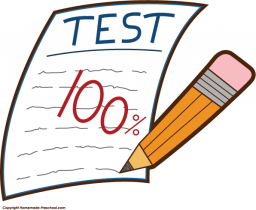# Probability 67264

The teacher has 20 questions, from which the student chooses two on the exam. The student learned 10 questions well, 6 partially, and 4 not at all. What is the probability that he will get both questions he knows well?

p =  0.2368

### Step-by-step explanation:Did you find an error or inaccuracy? Feel free to write us. Thank you!

Tips for related online calculators
Need help calculating sum, simplifying, or multiplying fractions? Try our fraction calculator.
Would you like to compute the count of combinations?